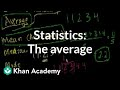Course

5

(21)

Certificate

This course is free today and always will be, from start to finish you will pay nothing.Teacher

Khan Academy is a 501(c)(3) nonprofit organization with the mission of providing a free, world-class education for anyone, anywhere. Our interactive practice problems, articles, and videos help students succeed in math, biology, chemistry, physics, history, economics, finance, grammar, and many other topics.

Course content preview

0h12m

0h06m

0h12m

0h11m

0h13m

0h12m

0h12m

0h10m

0h12m

0h11m

0h13m

0h10m

0h14m

0h16m

0h11m

0h12m

0h09m

0h26m

0h26m

ck12.org normal distribution problems: Qualitative sense of normal distributions | Khan Academy

0h10mWatch the 1st video of the course

Do not worry, it is free!

Course content0h12m

Statistics: The average | Descriptive statistics | Probability and Statistics | Khan Academy

Introduction to descriptive statistics and central tendency. Ways to measure the average of a set: median, mean, mode

Missed the previous lesson?

Probability and statistics on Khan Academy: We dare you to go through a day in which you never consider or use probability. Did you check the weather forecast? Busted! Did you decide to go through the drive through lane vs walk in? Busted again! We are constantly creating hypotheses, making predictions, testing, and analyzing. Our lives are full of probabilities! Statistics is related to probability because much of the data we use w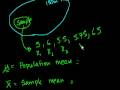0h06m

Statistics: Sample vs. Population Mean

The difference between the mean of a sample and the mean of a population.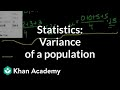0h12m

Statistics: Variance of a population | Probability and Statistics | Khan Academy

Variance of a population.

Missed the previous lesson?

Probability and statistics on Khan Academy: We dare you to go through a day in which you never consider or use probability. Did you check the weather forecast? Busted! Did you decide to go through the drive through lane vs walk in? Busted again! We are constantly creating hypotheses, making predictions, testing, and analyzing. Our lives are full of probabilities! Statistics is related to probability because much of the data we use when determining probable outcomes comes from our understanding of statistics. In these tutorials, we will cover a range of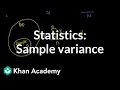0h11m

Statistics: Sample variance | Descriptive statistics | Probability and Statistics | Khan Academy

Using the variance of a sample to estimate the variance of a population

Missed the previous lesson?

Probability and statistics on Khan Academy: We dare you to go through a day in which you never consider or use probability. Did you check the weather forecast? Busted! Did you decide to go through the drive through lane vs walk in? Busted again! We are constantly creating hypotheses, making predictions, testing, and analyzing. Our lives are full of probabilities! Statistics is related to probability because much of the data we use when determining probable outcomes comes from our understanding o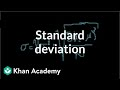0h13m

Statistics: Standard deviation | Descriptive statistics | Probability and Statistics | Khan Academy

Review of what we've learned. Introduction to the standard deviation.

Practice this lesson yourself on KhanAcademy.org right now:

Missed the previous lesson?

Probability and statistics on Khan Academy: We dare you to go through a day in which you never consider or use probability. Did you check the weather forecast? Busted! Did you decide to go through the drive through lane vs wal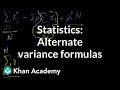0h12m

Statistics: Alternate variance formulas | Probability and Statistics | Khan Academy

Playing with the formula for variance of a population.

Missed the previous lesson?

Probability and statistics on Khan Academy: We dare you to go through a day in which you never consider or use probability. Did you check the weather forecast? Busted! Did you decide to go through the drive through lane vs walk in? Busted again! We are constantly creating hypotheses, making predictions, testing, and analyzing. Our lives are full of probabilities! Statistics is related to probability because much of the data we use when determining probable outcomes comes from our understanding of statistics.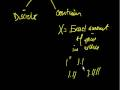0h12m

Introduction to Random Variables

Introduction to random variables and probability distribution functions.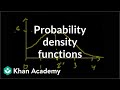0h10m

Probability density functions | Probability and Statistics | Khan Academy

Probability density functions for continuous random variables.

Missed the previous lesson?

Probability and statistics on Khan Academy: We dare you to go through a day in which you never consider or use probability. Did you check the weather forecast? Busted! Did you decide to go through the drive through lane vs walk in? Busted again! We are constantly creating hypotheses, making predictions, testin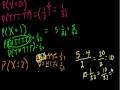0h12m

Binomial Distribution 1

Introduction to the binomial distribution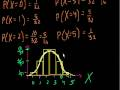0h11m

Binomial Distribution 2

More on the binomial distribution0h13m

Binomial Distribution 3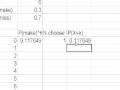0h10m

Binomial Distribution 4

Using Excel to visualize the basketball binomial distribution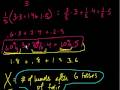0h14m

Expected Value: E(X)

Expected value of a random variable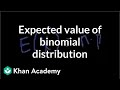0h16m

Expected value of binomial distribution | Probability and Statistics | Khan Academy

Missed the previous lesson?

Probability and statistics on Khan Academy: We dare you to go through a day in which you never consider or use probability. Did you check the weather forecast? Busted! Did you decide to go through the drive through lane vs walk in? Busted again! We are constantly creating hypotheses, making predictions, testing, and analyzing. Our lives are full of probabilities! Statistics is related to probability because much of the data we use when determining probable outcomes comes from our understanding of statistics. In these tutorials, we will cover a range of topics, some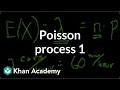0h11m

Poisson process 1 | Probability and Statistics | Khan Academy

Introduction to Poisson Processes and the Poisson Distribution.

Missed the previous lesson?

Probability and statistics on Khan Academy: We dare you to go through a day in which you never consider or use probability. Did you check the weather forecast? Busted! Did you decide to go through the drive through lane vs walk in? Busted again! We are constantly creating hypotheses, making predictions, testing, and analyzing. Our lives are full of probabilities! Statistics is related to probability because much of the data we use when determining probable outcomes comes from our understanding of statist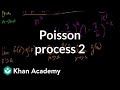0h12m

Poisson process 2 | Probability and Statistics | Khan Academy

More of the derivation of the Poisson Distribution.

Missed the previous lesson?

Probability and statistics on Khan Academy: We dare you to go through a day in which you never consider or use probability. Did you check the weather forecast? Busted! Did you decide to go through the drive through lane vs walk in? Busted again! We are constantly creating hypotheses, making predictions, testing, and analyzing. Our lives are full of probabilities! Statistics is related to probability because much of the data we use when determining probable outcomes comes from our understanding of statistics. In these tutorials, we will co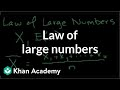0h09m

Law of large numbers | Probability and Statistics | Khan Academy

Introduction to the law of large numbers

Missed the previous lesson?

Probability and statistics on Khan Academy: We dare you to go through a day in which you never consider or use probability. Did you check the weather forecast? Busted! Did you decide to go through the drive through lane vs walk in? Busted again! We are constantly creating hypotheses, making predictions, testing, and analyzing. Our lives are full of probabilities! Statistics is related to probability because much of the data we use when determining probable outcomes comes from our understanding of statistics. In these tutorials, we will co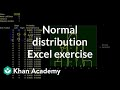0h26m

Normal distribution excel exercise | Probability and Statistics | Khan Academy

(Long-26 minutes) Presentation on spreadsheet to show that the normal distribution approximates the binomial distribution for a large number of trials.

Missed the previous lesson?

Probability and statistics on Khan Academy: We dare you to go through a day in which you never consider or use probability. Did you check the weather forecast? Busted! Did you decide to go through the drive through lane vs walk in? Busted again! We are constantly creating hypotheses, making predictions, testing, and analyzing. Our lives are full of probab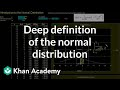0h26m

Introduction to the normal distribution | Probability and Statistics | Khan Academy

Exploring the normal distribution

Missed the previous lesson?

Probability and statistics on Khan Academy: We dare you to go through a day in which you never consider or use probability. Did you check the weather forecast? Busted! Did you decide to go through the drive through lane vs walk in? Busted again! We are constantly creating hypotheses, making predictions, testing, and analyzing. Our lives are full of probabilities! Statistics is related to probability because much of the data we use when determining probable outcomes comes from our understanding of statistics. In these tutorials,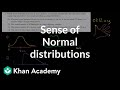0h10m

ck12.org normal distribution problems: Qualitative sense of normal distributions | Khan Academy

Discussion of how "normal" a distribution might be

Practice this lesson yourself on KhanAcademy.org right now: